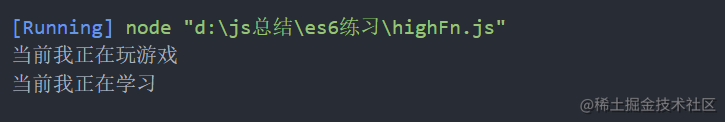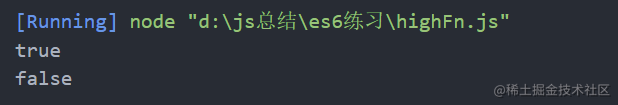# 高阶函数理解

## 高级函数概念理解

1. 一个函数的参数为函数
2. 一个函数返回值为函数

### 高阶函数

``````function study(...args) {
console.log('当前我正在' + args)
}

``````Function.prototype.before = function (beforeFn) {
return (...args) => {
beforeFn();
this(...args)
}
}

const play = (...args) => {
console.log('当前我正在' + args)
}

``````let newFn = study.before(() => {
play('玩游戏')
})

newFn('学习')## 柯理化函数

``````//原函数
return a+b+c
}

//柯理化函数
return function(b){
return function(c){
return a+b+c
}
}
}
//调用柯理化函数(充分利用了闭包的特性实现)

### 实现通用性函数

``````function currying(fn, args) {
//获取形参个数，即fn正常运行时所需参数的长度
let fnLen = fn.length;
//收集函数所传其他参数
args = args || []
return function () {
//将参数转化为数组
let _args = [].slice.call(arguments)
//将上次的参数与当前参数进行合并
Array.prototype.unshift.apply(_args, args);
//可替换成  _args = [...args, ..._args];
//把args数组加入到_args数组的前面，注意执行顺序

//参数不够则继续收集参数
if (_args.length < fnLen) {
return currying.call(null, fn, _args)
}
//如果参数满足，则直接执行
return fn.apply(null, _args)
}
}

``````//判断类型
function isType(type, value) {
return Object.prototype.toString.call(value) === `[object \${type}]`
}
let isString = curry(isType)('String');
let isNumber = curry(isType)('Number');
console.log(isString('abc'));
console.log(isNumber('abc'));